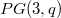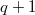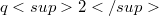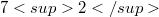﻿

### Spreads in$PG(3,q)$ admitting several homology groups of order$q+1$

#### Abstract

The set of translation planes with spreads in$PG(3,q)$ admitting at least three homology groups with distinct axes of order$q+1$ is completely determined. Apart from the Desarguesian and Hall planes of order$q2$, the only possible plane is the Heimbeck plane of order$72$ admitting several quaternion homology groups of order 8. A classification is also given of all translation planes with spreads in$PG(3,q)$ that admit at least three distinct homology groups of order$q+1$. Recent results conneting translation planes with spreads in$PG(3,q)$ admitting cyclic affine homology groups of order$q+1$ with conical flocks spreads provide the background for applications showing how the associated collineation groups are interrelated.

DOI Code: 10.1285/i15900932v24n2p9

Keywords: Homology groups; Translation planes; Spreads; Flocks of quadratic cones; Hyperbolic fibrations

Classification: 51E23; 51A40

Full Text: PDF